# On-Line Ohba's Conjecture

 Importance: Medium ✭✭
 Author(s): Huang, Po-Yi Wong, Tsai-Lien Zhu, Xuding
 Subject: Graph Theory » Coloring » » Vertex coloring
 Keywords: choosability list coloring on-line choosability
 Recomm. for undergrads: yes
 Posted by: Jon Noel on: April 11th, 2013
Conjecture   If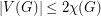, then the on-line choice number ofis equal to.

The on-line choice number of a graphis determined by the following-player game. We call the players Painter and Lister. Letbe an infinite set of colours.

Initially, every vertexofis given a listof colours. At theth step of the game, Lister chooses a non-empty set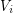of vertices which are not coloured and, for each vertex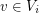, the list ofis changed to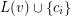. Then, painter chooses a stable subset ofto colour with.

If, at some step of the game, there is a vertexsuch that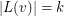andis not coloured with, then Lister wins the game. Otherwise, if every vertex is eventually coloured, then Painter wins. The on-line choice number of, denoted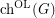is defined to be the minimumsuch that Painter has a winning strategy.

The on-line choice number was introduced independently by Schauz [Sch09] and Zhu [Zhu09].

It is clear that the on-line choice number is at least as large as the choice number; ie.. That is, if there is an assignmentof lists of sizesuch that there is no acceptable colouring, then Lister can simply play so that the resulting lists are given by.

For choosability, Ohba [Ohb02] made the following conjecture, which was proved by Noel, Reed and Wu [NRW12]: if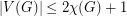, then TeX Embedding failed!.}

The On-Line Ohba's Conjecture, proposed by Huang, Wong and Zhu [HWZ12], assumes a stronger bound on the number of vertices (namely,). A result of Kim, Kwon, Liu and Zhu [KKLZ12] is that, for, the complete-partite graph with one part of sizeand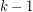parts of sizehas on-line choice number. Therefore, On-Line Ohba's Conjecture cannot be extended to graphs on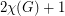vertices.

In [KSW14], it is proved that the complete-partite graph with parts of sizehas choice number, but on-line choice number at least. It is also claimed that a computer check shows thatis the correct value for the on-line choice number (no formal proof is given).

Some partial results are known:

Theorem  (Huang et al. 2012)   The on-line choice number of the complete-partite graph with all parts of sizeis.
Theorem  (Kozik, Micek, Zhu 2012)   On-Line Ohba's Conjecture is true for graphs of independence number at most.
Theorem  (Kozik et al. 2012)   If, then.
Theorem  (Carraher et al. 2012)   If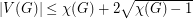, then.

Presently, it is not known whether there is a real constant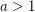such that if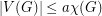, then.

## Bibliography

[CLM+13] J. Carraher, S. Loeb, T. Mahoney, G. Puleo, M.-T. Tsai, and D. West. Three Topics in Online List Coloring. Preprint, February 2013.

*[HWZ12] P. Huang, T. Wong, and X. Zhu. Application of polynomial method to on-line list colouring of graphs. European J. Combin., 33(5):872–883, 2012.

[KSW14] H. A. Kierstead, A. Salmon and R. Wang. On the Choice Number of Complete Multipartite Graphs With Part Size Four.

[KKLZ12] S.-J. Kim, Y. S. Kwon, D. D.-F. Liu, and X. Zhu. On-line list colouring of complete multipartite graphs. Electron. J. Combin., 19(1):Paper 41, 13, 2012.

[KMZ12] J. Kozik, P. Micek, and X. Zhu. Towards on-line Ohba’s conjecture. Preprint, arXiv:1111.5458v2, December 2012.

[Noe13] J. A. Noel. Choosability of Graphs With Bounded Order: Ohba's Conjecture and Beyond. Master's thesis, McGill University, Montreal. pdf

[NRW12] J. A. Noel, B. A. Reed, and H. Wu. A Proof of a Conjecture of Ohba. Preprint, arXiv:1211.1999v1, November 2012. Webpage

[Ohb02] K. Ohba. On chromatic-choosable graphs. J. Graph Theory, 40(2):130–135, 2002.

[Sch09] U. Schauz. Mr. Paint and Mrs. Correct. Electron. J. Combin., 16(1):Research Paper 77, 18, 2009.

[Sch10] U. Schauz. A paintability version of the combinatorial Nullstellensatz, and list colorings of k-partite k-uniform hypergraphs. Electron. J. Combin., 17(1):Research Paper 176, 13, 2010.

[Zhu09] X. Zhu. On-line list colouring of graphs. Electron. J. Combin., 16(1):Research Paper 127, 16, 2009.

* indicates original appearance(s) of problem.